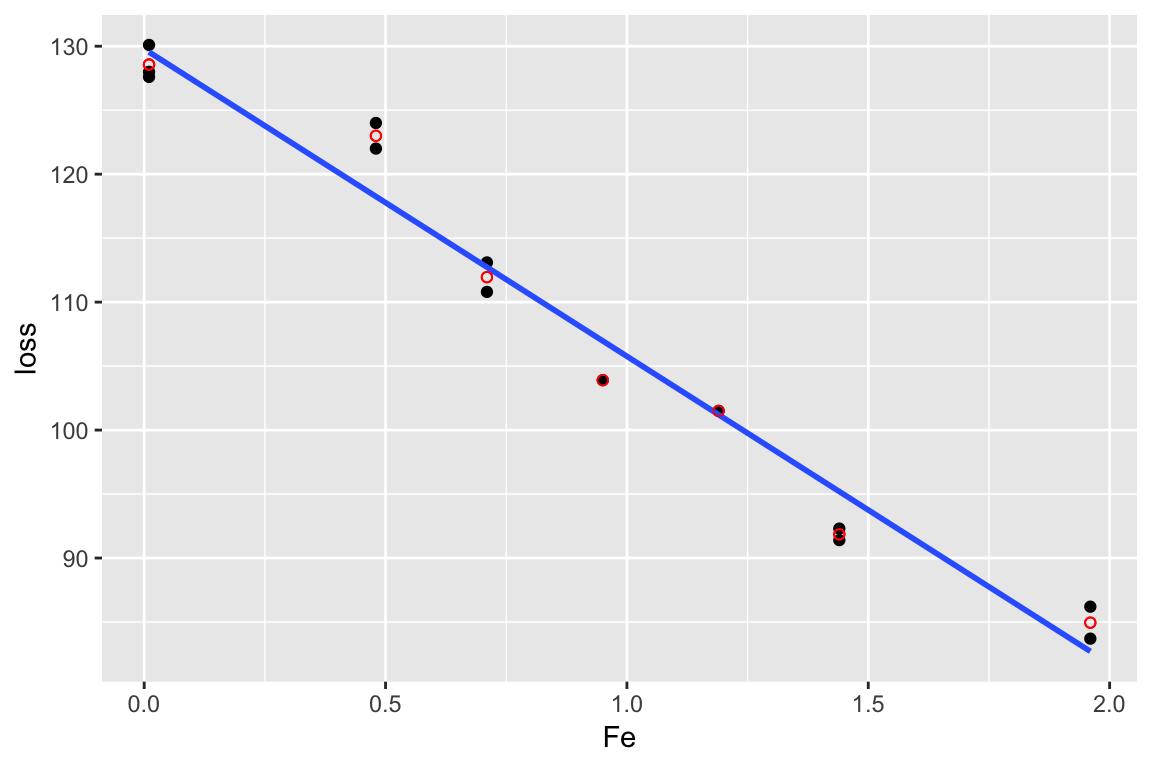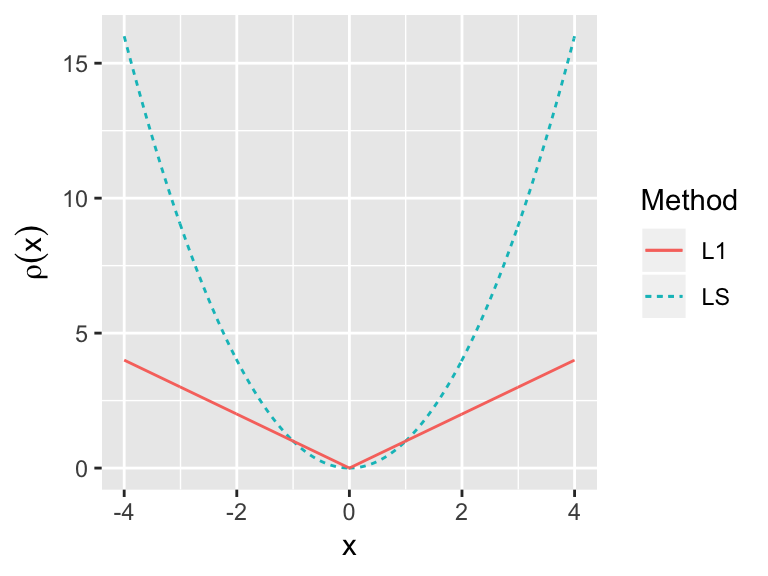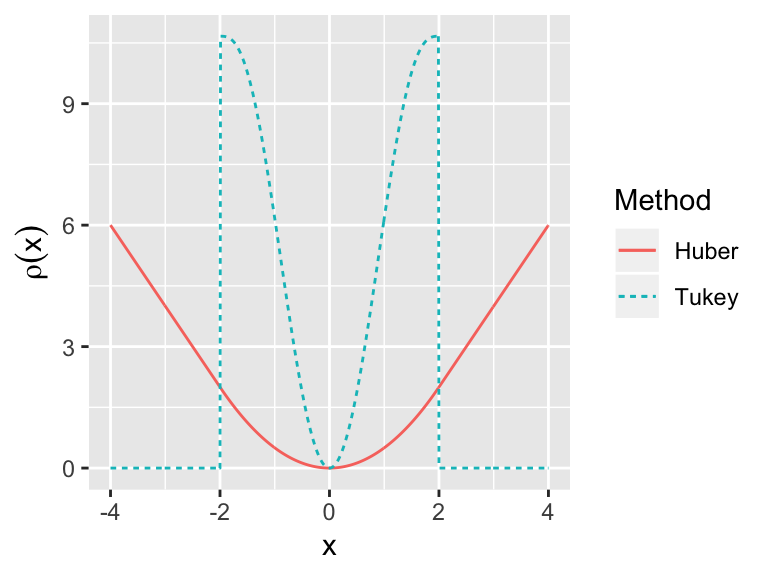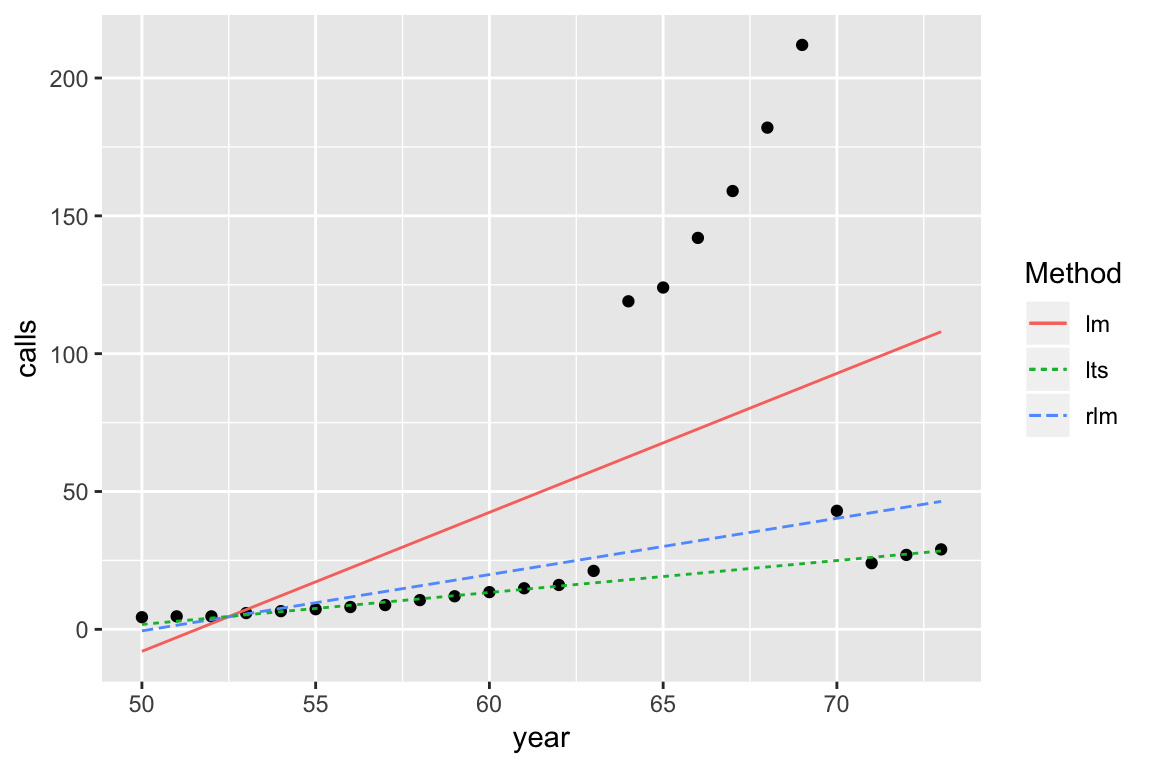# Problems with the errorFeb 22 2019

## Today

Problems with the errors

• Generalized Least Squares
• Lack of fit F-tests
• Robust regression

## Generalized Least Squares

$Y = X\beta + \epsilon$

• We have assumed $$\Var{\epsilon} = \sigma^2 I$$, but what if we know $$\Var{\epsilon} = \sigma^2 \Sigma$$, where $$\sigma^2$$ is unknown, but $$\Sigma$$ is known. For example, we know the form of the correlation and/or non-constant variance in the response.

• The usual least squares estimates $$\hat{\beta}_{LS}$$ are unbiased, but they are no longer BLUE.

Let $$S$$ be the matrix square root of $$\Sigma$$, i.e. $$\Sigma = SS^T$$.

Define a new regression equation by multiplying both sides by $$S^{-1}$$: \begin{aligned} S^{-1}Y &= S^{-1}X\beta + S^{-1}\epsilon \\ Y' &= X' \beta + \epsilon' \end{aligned}

Show $$\Var{\epsilon'} = \Var{S^{-1}\epsilon} = \sigma^2 I$$.

Show the least squares estimates for the new regression equation reduce to: $\hat{\beta} = (X^T\Sigma^{-1} X)^{-1}X^T\Sigma^{-1} Y$

• Can also show $$\Var{\beta} = (X^T\Sigma^{-1} X)^{-1} \sigma^2$$.

• The estimates: $$\hat{\beta} = (X^T\Sigma^{-1} X)^{-1}X^T\Sigma^{-1} Y$$ are known as estimates.

• In practice, $$\Sigma$$ might only be know up to a few parameters that also need to be estimated.

## Common cases of GLS

• $$\Sigma$$ defines a temporal or spatial correlation structure
• $$\Sigma$$ defines a grouping structure
• $$\Sigma$$ is diagonal and defines a weighting structure ()

## In R

?lm # use weights argument
library(nlme)
?gls # has weights and/or correlation argument

## Oat yields

Data from an experiment to compare 8 varieties of oats. The growing area was heterogeneous and so was grouped into 5 blocks. Each variety was sown once within each block and the yield in grams per 16ft row was recorded.

$\text{yield}_i = \beta_0 + \beta_1 \text{variety}_i + \epsilon_i \quad i=1, \ldots, 40$ $\Var{\epsilon_i} = \sigma^2, \quad \text{Cor}(\epsilon_i, \epsilon_j) = \begin{cases} \rho, & \text{block}_i = \text{if block}_j \\ 0, & \text{otherwise} \end{cases}$

library(nlme)
fit_gls <- gls(yield ~ variety, data = oatvar,
correlation = corCompSymm(form = ~ 1 | block))

## Oat yields in R

intervals(fit_gls)
## Approximate 95% confidence intervals
##
##  Coefficients:
##                  lower  est.       upper
## (Intercept) 291.542999 334.4 377.2570009
## variety2     -4.903898  42.2  89.3038984
## variety3    -18.903898  28.2  75.3038984
## variety4    -94.703898 -47.6  -0.4961016
## variety5     57.896102 105.0 152.1038984
## variety6    -50.903898  -3.8  43.3038984
## variety7    -63.103898 -16.0  31.1038984
## variety8      2.696102  49.8  96.9038984
## attr(,"label")
##  "Coefficients:"
##
##  Correlation structure:
##          lower      est.     upper
## Rho 0.06596382 0.3959955 0.7493731
## attr(,"label")
##  "Correlation structure:"
##
##  Residual standard error:
##    lower     est.    upper
## 33.39319 47.04679 66.28298

## Lack of fit F-tests

• $$\hat{\sigma^2}$$ should be (if our model is specified correctly) an unbiased estimate of $$\sigma^2$$.

• A “model free” estimate of $$\sigma^2$$ is available if there are replicates (multiple observations at combinations of the explanatory values).

• If our $$\hat{\sigma^2}$$ from our model is much bigger than the “model-free” estimate, we have evidence of .

## In practice

• Fit a saturated model. Compare saturated model to proposed model with an F-test. .

• Saturated: every combination of explanatory variables is allowed its own mean (i.e. every group of replicates is allowed its own mean). A model that includes every explantory as categorical and every possible interaction between variables.

## Example

data(corrosion, package = "faraway")
lm_cor <- lm(loss ~ Fe, data = corrosion)
lm_sat <- lm(loss ~ factor(Fe), data = corrosion)
anova(lm_cor, lm_sat)
## Analysis of Variance Table
##
## Model 1: loss ~ Fe
## Model 2: loss ~ factor(Fe)
##   Res.Df     RSS Df Sum of Sq      F   Pr(>F)
## 1     11 102.850
## 2      6  11.782  5    91.069 9.2756 0.008623 **
## ---
## Signif. codes:  0 '***' 0.001 '**' 0.01 '*' 0.05 '.' 0.1 ' ' 1
# significant lack of fit## Robust regression

Remember to define our least squares estimates we looked for $$\beta$$ to minimise $\sum_{i=1}^n \left(y_i - x_i^T\beta \right)^2$

In practice, since we are squaring residuals, observations with large residuals carry a lot of weight. For, robust regression, we want to downweight the observations with large residuals.

The idea of M-estimators is to extend this to the general situation where we want to find $$\beta$$ to minimise $\sum_{i=1}^n \rho(y_i - x_i^T\beta)$ where $$\rho()$$ is some function we specify.$\sum_{i=1}^n \rho(y_i - x_i^T\beta)$

• Least squares: $$\rho(e_i) = e_i^2$$
• Least absolute deviation, L$$_1$$ regression: $$\rho(e_i) = |e_i|$$
• Huber’s method $\rho(e_i) = \begin{cases} e_i^2/2 & \text{if } |e_i| \le c\\ c|e_i| - c^2/2 & \text{otherwise} \end{cases}$
• Tukey’s bisquare $\rho(e_i) =\begin{cases} \frac{1}{6}(c^6 - (c^2 - e_i^2)^3) & |e_i| \le c\\ 0 & \text{otherwise} \end{cases}$

The models are usually fit in an iterative process.

## Least trimmed squares

Minimise the smallest residuals $\sum_{i=1}^q e_{(i)}^2$ where $$q$$ is some number smaller than $$n$$ and $$e_{(i)}$$ is the ith smallest residual.

One choice, $$q=\lfloor n/2 \rfloor + \lfloor (p+1)/2 \rfloor$$

Annual numbers of telephone calls in Belgium